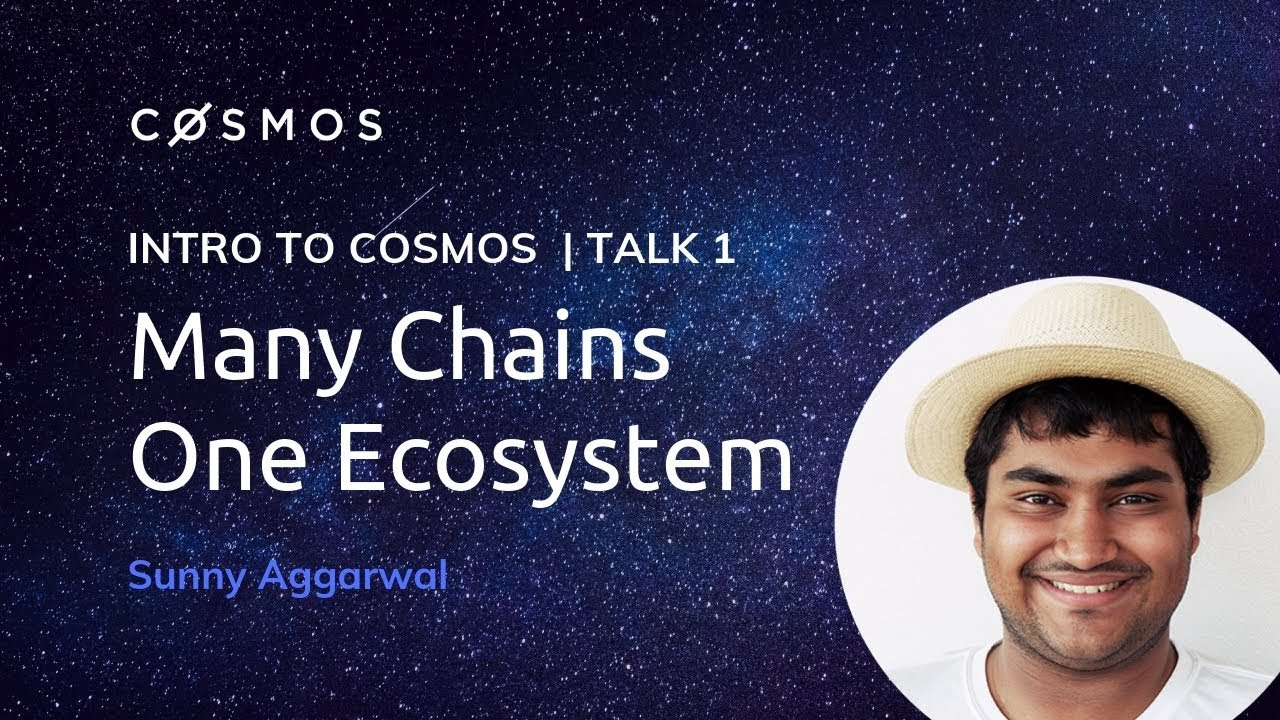# Intro to the Cosmos Network | Sunny AggarwalSunny Aggarwal provides an intro to Cosmos.

MORE LINKS Tendermint BFT Formal specification with proofs of safety and liveness: https://arxiv.org/abs/1807.04938 Tendermint Diagram: http://bit.ly/2Nfl9Vb Casper vs Tendermint: https://bit.ly/2Iu4Uno

Tendermint Core Tendermint Core Docs: https://tendermint.com/docs/ Performance Testing Results: https://bit.ly/2NKCW9n Ethan Buchman’s Masters Thesis: https://bit.ly/2S9PyoF

## Neural network: what is a neural network?

Neural networks, as their name implies, are computer algorithms modeled after networks of neurons in the human brain. Learn more about neural networks from Algorithmia.

## A Comparative Analysis of Recurrent Neural Networks

Recurrent neural networks, also known as RNNs, are a class of neural networks that allow previous outputs to be used as inputs while having hidden states.

## Recurrent Neural Networks for Multilabel Text Classification Tasks

The purpose of this project is to build and evaluate Recurrent Neural Networks(RNNs) for sentence-level classification tasks. Let's understand about recurrent neural networks for multilabel text classification tasks.

## Neural Network on Beer Dataset

Artificial neural networks (ANNs), usually simply called neural networks (NNs), are computing systems vaguely inspired by the biological neural networks that constitute animal brains.

## Convolutional Neural Network: How is it different from the other networks?

Convolutional Neural Network: How is it different from the other networks? What’s so unique about CNNs and what does convolution really do? This is a math-free introduction to the wonders of CNNs.